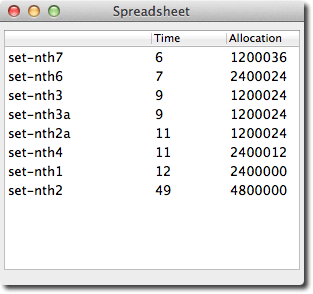## Changing the nth item of a list

17th August 2015

Some time ago I posted this question on StackExchange :

### The question

I want change the nth element of a list and return a new list.

I've thought of three rather inelegant solutions:

```(defun set-nth1 (list n value)
(let ((list2 (copy-seq list)))
(setf (elt list2 n) value)
list2))```
```(defun set-nth2 (list n value)
(concatenate 'list (subseq list 0 n) (list value) (subseq list (1+ n))))```
```(defun set-nth3 (list n value)
(substitute value nil list
:test #'(lambda (a b) (declare (ignore a b)) t)
:start n
:count 1))```

What is the best way of doing this?

The responses

To clarify, I wanted an answer that didn't alter the original list, and I preferred answers that were intuitive over answers that were obscure.

There were some interesting comments and suggestions. Terge Norderhaug and user 6502 suggested a variant of set-nth2 using nconc rather than concatenate, and nthcdr to reuse the tail of the original list:

```(defun set-nth2a (list n val)
(nconc (subseq list 0 n)
(cons val (nthcdr (1+ n) list))))```

User huaiyuan pointed out that there is a function constantly  which can be used as the test in set-nth3:

```(defun set-nth3a (list n value)
(substitute value nil list
:test (constantly t)
:start n
:count 1))```

User huaiyuan suggested this version using fill:

```(defun set-nth4 (list n value)
(fill (copy-seq list) value :start n :end (1+ n)))```

User 6502 suggested the following recursive definition:

```(defun set-nth5 (list n val)
(if (> n 0)
(cons (car list)
(set-nth5 (cdr list) (1- n) val))
(cons val (cdr list))))```

### Loop macro

Finally, there were a couple of suggestions using the loop macro. User huaiyuan suggested:

```(defun set-nth6 (list n val)
(loop for i from 0 for j in list collect (if (= i n) val j)))```

and user WReach suggested this solution:

```(defun set-nth7 (list n value)
(loop
for cell on list
for i from 0
when (< i n) collect (car cell)
else collect value
and nconc (rest cell)
and do (loop-finish)))```

### Which is best?

So now there are eight alternatives; I was interested to see how they would compare in terms of execution time and memory allocation. To do this I used a Spreadsheet application  using LispWorks's CAPI interface to run execution time and memory allocation benchmarks for each version of set-nth, and then display the results in a table.

First I created a list of 200000 pairs as dummy test data:

```(defparameter *test-data*
(let (result)
(dotimes (n 200000)
(let ((string (format nil "~r" n)))
(push (cons string (length string)) result)))
(nreverse result)))```

Here's the benchmark program, which replaces the 100000th pair with a new value:

```(defun measure (fn value)
(let (start end (best most-positive-fixnum))
(dotimes (x 10 best)
(cond
;; Get the run time
((eq value 'time)
(setq start (get-internal-run-time))
(funcall fn *test-data* 100000 '(("fish" . 4)))
(setq end (get-internal-run-time))
(setq best (min best (- end start))))
;; Get the memory allocated
((eq value 'allocation)
(setq start (total-allocation))
(funcall fn *test-data* 100000 '(("fish" . 4)))
(setq end (total-allocation))
(setq best (min best (- end start)))))))))```

For example, to get the time benchmark for set-nth1 you run:

`(measure 'set-nth1 'time)`

The routine takes the minimum value of 10 measurements, to try and get the best estimate.

Finally I ran my spreadsheet function with a list of the functions to be compared :

```(spreadsheet
'(set-nth1 set-nth2 set-nth2a set-nth3 set-nth3a set-nth4 set-nth6 set-nth7)
'(time allocation) #'measure
:row-label-fn #'string-downcase :column-label-fn #'string-capitalize)```

This displays the benchmarks in a table. Sorting by the Time column gives:The best version is set-nth7, which uses the loop macro. Of the other versions set-nth3 and set-nth3a using substitute are the best, both by time and memory allocation, and to my mind they're also the most intuitive.

The worst version is set-nth2 which uses concatenate. It allocates four times as much memory and takes nearly 8 times longer than the best version!

1. ^ Changing the nth element of a list on StackOverflow.
2. ^ CONSTANTLY in the Common Lisp HyperSpec.
3. ^ Spreadsheet on CAPI Cookbook.
4. ^ I couldn't include set-nth5 because it caused a stack overflow.